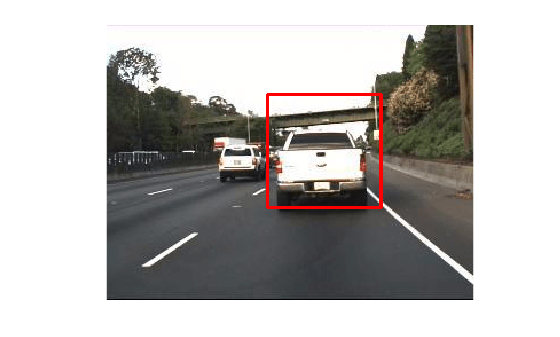# rectToBbox

Compute bounding boxes from outputs returned by MATLAB interface object for OpenCV `Rect` class

## Syntax

``bbox = rectToBbox(input)``

## Description

example

````bbox = rectToBbox(input)` computes bounding box values from the outputs returned by a MATLAB® interface object for OpenCV `Rect` class. The OpenCV `Rect` class creates rectangles on an input image. This function maps the zero-based indexing in OpenCV to one-based indexing in MATLAB.```

## Examples

collapse all

Draw a rectangle on an image by using the prebuilt MATLAB interface for the OpenCV function `cv::rectangle`. Compute the corresponding bounding box values in MATLAB to use to crop the image region.

Add the MATLAB interface to OpenCV package names to the import list.

```import clib.opencv.*; import vision.opencv.util.*;```

Read an image into the MATLAB workspace.

`img = imread("highway.png");`

Create interface objects for the OpenCV `Mat` and `InputOutputArray` classes to store the input image.

`[inputMat,ocvArray] = createMat(img,"InputOutput");`

Define Rectangular Region

Call the OpenCV function `cv::Rect2i` using MATLAB, and specify the coordinates and dimensions for the rectangle. Display the values.

`rec = cv.Rect2i(140,60,100,100)`
```rec = Rect2i with properties: x: 140 y: 60 width: 100 height: 100 ```

Draw Rectangle and Display Results

Specify the properties of the line to use for drawing the rectangle. Set these values:

• Color of the line to red. To set this value, use the OpenCV function `cv::Scalar`.

• Thickness of the line to `2`.

• Line type to `4`.

• Shift to `0`.

```color = cv.Scalar(255,0,0); thickness = 2; lineType = 4; shift = 0;```

Draw the defined rectangle on the image by using the OpenCV function `cv::rectangle`.

`cv.rectangle(ocvArray,rec,color,thickness,lineType,shift);`

Read and display the output image.

```outputImg = getImage(ocvArray); figure imshow(outputImg)```Compute Bounding Box

Use the utility function `rectToBbox` to compute the bounding box value from the values returned by OpenCV function `cv::Rect2i`.

`bbox = rectToBbox(rec);`

Display the bounding box values. Notice that the bounding box values in the MATLAB workspace have one-based indexing.

`bbox`
```bbox = 1x4 int32 row vector 141 61 100 100 ```

Crop the region within the bounding box by using the `imcrop` function and display the cropped image.

```croppedImg = imcrop(img,bbox); figure imshow(croppedImg)```## Input Arguments

collapse all

OpenCV class for rectangles, specified as one of these values:

• `Rect__unsignedChar_` interface object — This MATLAB interface object is a representation of the OpenCV class `cv::Rect_<unsigned char>`.

• `Rect2d` interface object — This MATLAB interface object is a representation of the OpenCV class `cv::Rect_<double>`.

• `Rect2f` interface object — This MATLAB interface object is a representation of the OpenCV class `cv::Rect_<float>`.

• `Rect2i` interface object — This MATLAB interface object is a representation of the OpenCV class `cv::Rect_<int>`.

• `Rect2i` interface object — This MATLAB interface object is a representation of the OpenCV class `cv::Rect_<int>`.

## Output Arguments

collapse all

Bounding box values, returned as a four-element row vector of the form [`x` `y` `width` `height`].